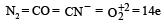Courses

# Test: Structure Of Atom 2 - From Past 28 Years Questions

## 30 Questions MCQ Test Chemistry Class 11 | Test: Structure Of Atom 2 - From Past 28 Years Questions

Description
This mock test of Test: Structure Of Atom 2 - From Past 28 Years Questions for NEET helps you for every NEET entrance exam. This contains 30 Multiple Choice Questions for NEET Test: Structure Of Atom 2 - From Past 28 Years Questions (mcq) to study with solutions a complete question bank. The solved questions answers in this Test: Structure Of Atom 2 - From Past 28 Years Questions quiz give you a good mix of easy questions and tough questions. NEET students definitely take this Test: Structure Of Atom 2 - From Past 28 Years Questions exercise for a better result in the exam. You can find other Test: Structure Of Atom 2 - From Past 28 Years Questions extra questions, long questions & short questions for NEET on EduRev as well by searching above.
QUESTION: 1

### The spectrum of He is expected to be similar to that

Solution:

Both He and Li+ contain 2 electrons each therefore their spectrum will be similar.

QUESTION: 2

### The number of spherical nodes in 3p orbitals are    

Solution:

No. of radial nodes in 3p-orbital = (n – ℓ – 1) [for p ortbital ℓ = 1] = 3 – 1 – 1 = 1

QUESTION: 3

### If r is the radius of the first orbit, the radius of nth orbit of H-atom is given by 

Solution:

Radius of nth orbit = r1 n2 . (for H-atom)

QUESTION: 4

Which of the following statements do not form a part of Bohr ’s model of hydrogen atom ? 

Solution:

is uncertainty principle which was given by Hiesenberg and not Bohr’s postulate

QUESTION: 5

Number of unpaired electrons in N2+ is 

Solution:

N(7) = 1s2 2s2 2p2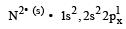Unpaired electrons = 1.

QUESTION: 6

The maximum number of electrons in a subshell is given by the expression 

Solution:

No. of orbitals in a sub-shell = 2 ℓ + 1

⇒ No. of electrons = 2(2ℓ + 1) = 4ℓ + 2

QUESTION: 7

The total number of electrons that can be accommodated in all the orbitals having principal quantum number 2 and azimuthal quantum number 1 is 

Solution:

n = 2, l = 1 means 2 p – orbital. Electrons that can be accommodated = 6 as p sub-shell has 3 orbital and each orbital contains 2 electrons.

QUESTION: 8

An ion has 18 electrons in the outermost shell, it is

Solution:

Cu+ = 29 – 1 = 28 e thus the electronic confingration of Cu+ is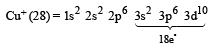QUESTION: 9

In a given atom no two electrons can have the same values for all the four quantum numbers.This is called 

Solution:

This is as per the definiti on of Paul i’s Exclusion princieple.

QUESTION: 10

For azimuthal quantum number ℓ = 3, the maximum number of electrons will be 

Solution:

ℓ = 3 means f-subshell. Maximum no. of electrons = 4ℓ + 2 = 4 × 3 + 2 = 14

QUESTION: 11

The order of filling of electrons in the orbitals of an atom will be 

Solution:

The sub-shell with lowest value of (n + ℓ) is filled up first. When two or more sub-shells have same (n + ℓ) value the subshell with lowest value of 'n' is filled up first therefore the correct order is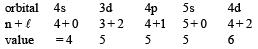QUESTION: 12

The energy of an electron in then the Bohr orbit of hydrogen atom is 

Solution:

Energy of an electron in Bohr's orbit is given by the relationship.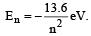QUESTION: 13

Which of the following species has four lone pairs of electrons?

Solution:

Lone pair of electron means 2 electron(since it is pair)
For 4 lone pair electrons, we need 8 electrons in the outer shell. This is possible only with Cl- as it neutral Cl has 7 electrons and with addition of an electron they are 8 in number or 4 lone pairs of electrons.

QUESTION: 14

If ionization potential for hydrogen atom is 13.6 eV, then ionization potential for He+ will be

Solution:

The ionization energy of any hydrogen like species (having one electron only) is given by the equation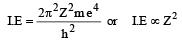Since the atomic number of H is 1 and that of He is 2, therefore, the I.E. of He+ is four times (22) the I.E. of H i.e., 13.6 × 4 = 54.4 eV

QUESTION: 15

For which one of the following sets of four quantium numbers, an electron will have the heighst energy?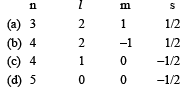Solution:

The sub-shell are 3d, 4d, 4p and 4s, 4d has highest energy as n + ℓ value is maximum for this.

QUESTION: 16

Which of the following is never true for cathode rays ?

Solution:

Cathode r ays are never electromagnetic waves.

QUESTION: 17

The electron was shown experimentally to have wave properties by 

Solution:

Wave nature of electr on was shown by Davisson and Germer. Davission and germer demonstrated the physical reality of the wave nature of electrons by showing that a beam of electrons could also diffracted by crystals just like light of x-rays.

QUESTION: 18

When an electron of charge e and mass m moves with a velocity v about the nuclear charge Ze is circular orbit of radius r, the potential energy of the electrons is given by 

Solution:

P.E. = work done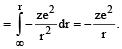QUESTION: 19

In the photo-electron emission, the energy of the emitted electron is 

Solution:

K.E. of emitted electron = hν - hν0 (i.e. smaller than hν ).

QUESTION: 20

If electron has spin quantum number  + 1/2 and a magnetic quantum number – 1, it cannot be present in 

Solution:

It cannot be present in s-orbital.

QUESTION: 21

The radius of hydrogen atom in the ground state is 0.53 Å. The radius of Li2+ ion (atomic number = 3) in a similar state is 

Solution:

State of hydrogen atom (n) = 1 (due to ground state) Radius of hydrogen atom (r) = 0.53 Å.
Atomic number of Li (Z) = 3.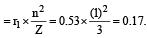QUESTION: 22

Uncertainty in position of an electron (mass = 9.1 × 10–28 g) moving with a velocity of 3 × 104 cm/s accurate upto 0.001% will be (use h/4π) in uncertainty expression where h  = 6.626 ×10–27 erg-second) 

Solution:

Given mass of an electron(m) = 9.1x10 -28g; Velocity of electron (v) = 3 x104cm/s;

Accuracy in velocity  = 0.001%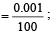Actual velocity of the electron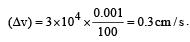.Planck’s constant (h) = 6.626×10–27erg-sec.

∴  Uncertainty in the position of the electron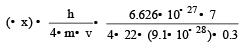=1.93cm

QUESTION: 23

The orbitals are called degenerate when 

Solution:

The orbitals which have same energy are called degenerate orbitals eg. px , py , pz .

QUESTION: 24

The momentum of a particle having a de Broglie wavelength of 10–17 metres is  (Given h = 6.625 × 10–34 Js)

Solution:

Acc. to deBroglie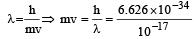.

⇒   p = 6.626 × 10–17 kg m/s

QUESTION: 25

The ion that is isoelectronic with CO is 

Solution:

We know that ions which have th e same number of electrons are called isoelectronic.
We also know that both CO and CNhave 14 electrons, therefore these are isoelectronic.

QUESTION: 26

The Bohr orbit radius for the hydrogen atom (n = 1) is approximately 0.530 Å. The radius for the first excited state (n = 2) orbit is (in Å) 

Solution:

Given : Radius of hydrogen atom = 0.530 Å,
Number of excited state (n) = 2 and atomic number of hydrogen atom (Z) = 1. We know that the Bohr radius.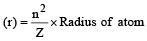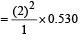= 4 x 0.530 = 2.12 Å

QUESTION: 27

The position of both , an electron and a helium atom is known within 1.0 nm. Further the momentum of the electron is known within 5.0 × 10–26 kg ms–1. The minimum uncertainty in the measurement of the momentum of the helium atom is 

Solution:

By Heisenberg uncertainty Principle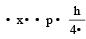(which is constant)

As Δx for electron and helium atom is same thus momentum of electron and helium will also be same therefore the momentum of helium atom is equal to 5 × 10–26 kg. m.s–1.

QUESTION: 28

According to Bohr ’s theory the energy required for an electron in the Li2+ ion to be emitted from n = 2 state is (given that the ground state ionization energy of hydrogen atom is 13.6 eV)

Solution:

Energy of electron in 2nd or bit of Li+2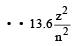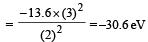Energy required = 0 – (–30.6) = 30.6 eV

QUESTION: 29

If the energy of a photon is given as : = 3.03 × 10–19 J then, the wavelength (λ) of the photon is :

Solution:

The energy of photon,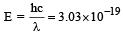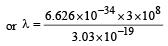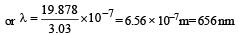QUESTION: 30

Set of isoelectronic species is 

Solution:

The molecule which contains same number of electrons are called isoelectronic. eg.